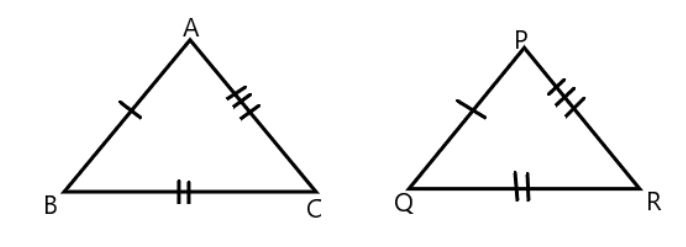Courses
Courses for Kids
Free study material
Offline Centres
MoreLast updated date: 23rd Nov 2023
Total views: 278.1k
Views today: 3.78k

# In the example given, a pair of triangles is shown. Equal parts of triangles in pairs are marked with the same signs. Observe the figures and state the test by which the triangles in pairs are congruent.Verified
Hint: In the above question we have two triangles and we have to find the congruence criteria. We know that we can say that the triangles are congruent when every corresponding side and interior angles are of the same length, though the triangles are of different size and shape. In the congruence of triangles we check the side and angle of triangles, so different criteria are $SAS,ASA,SSS$ and $AAS$ .
In the above given figure we have two triangle i.e. $\Delta ABC,\Delta PQR$ .
It is given that in the given pair of triangle Side $AB =$ Side $PQ$ . Similarly we have $BC = QR$ and the sides $AC = PR$ .
Now we know that if two triangles have all the three sides of one triangle and are of equal length of the three sides of the other triangle , then we can say that these triangles are congruent by $SSS$ criteria of $Side - Side - Side$ congruence.
Hence the required answer is that $\Delta ABC$ and $\Delta PQR$ are congruent by $SSS$ criteria.
So, the correct answer is “ $SSS$ ”.
Note: We should note that since we can be sure that the triangles are congruent then this suggests that the three angles of one triangle are equal to the angles of the other triangle respectively. We should know that there is one other criteria which is $RHS$ i.e. Right angle hypotenuse congruence. This rule means that the triangle must have right angles and if the hypotenuse and one side of a triangle measure the same as the hypotenuse and one side of another triangle, then the two pairs of triangle are congruent by $RHS$ criteria.